## 前向计算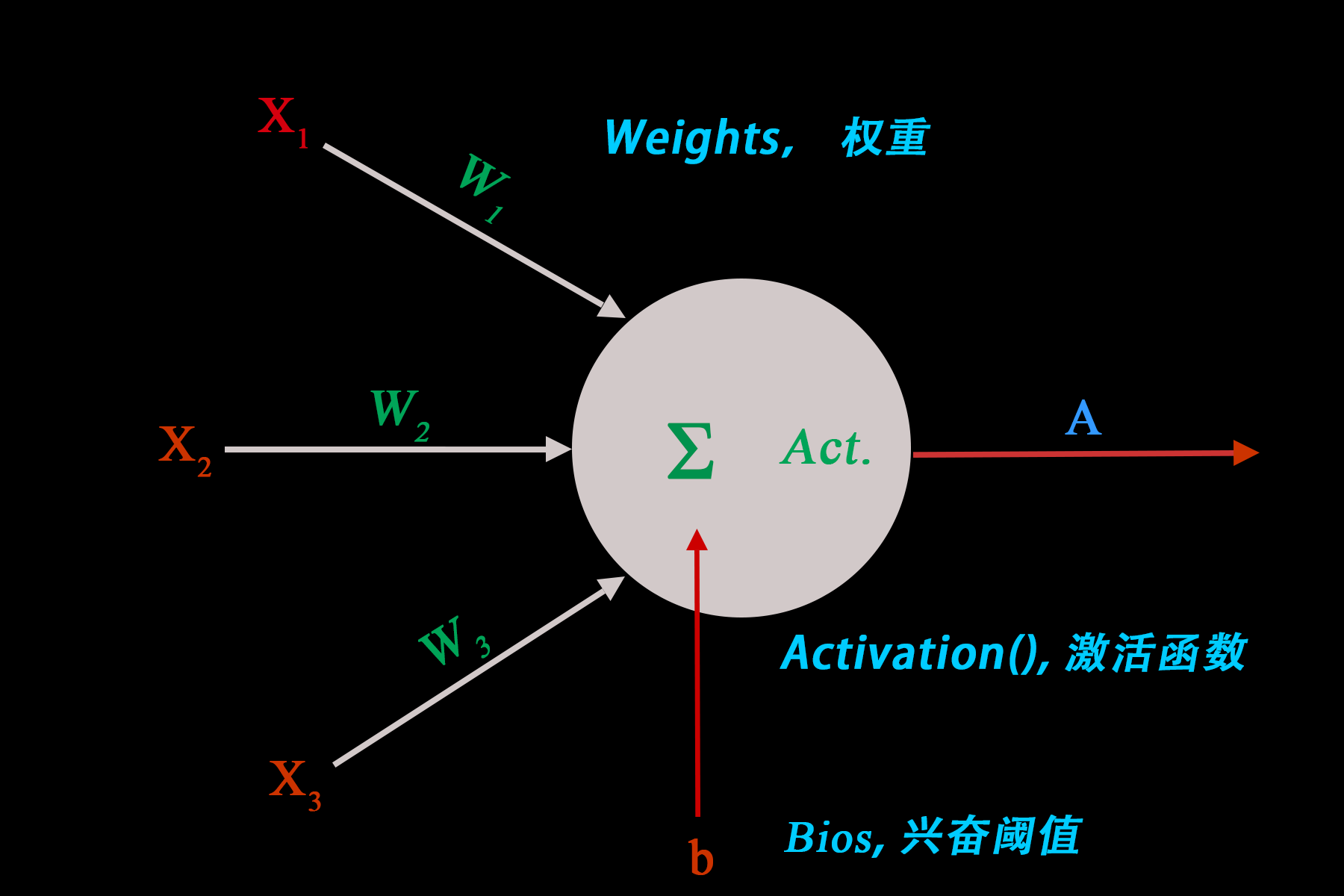$Z=\sum_{i=1}^{3}W_iX_i+b$$A=Activation(Z)$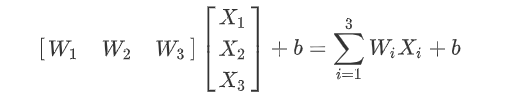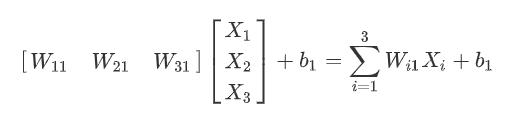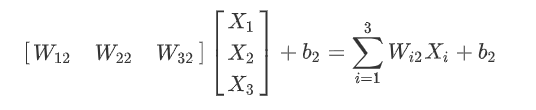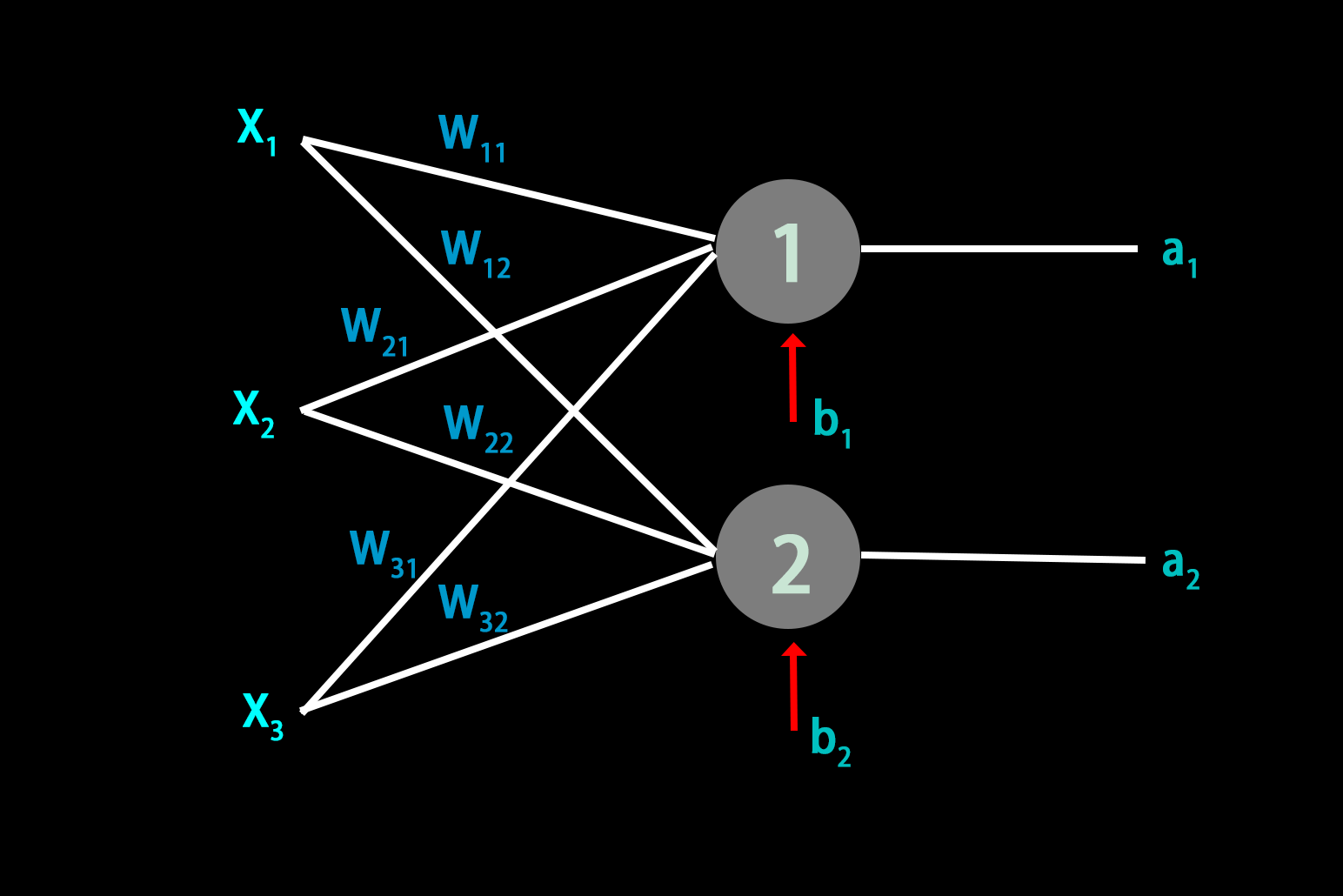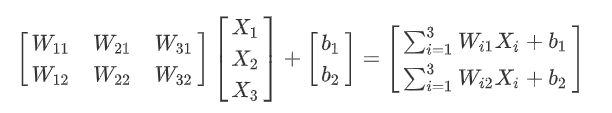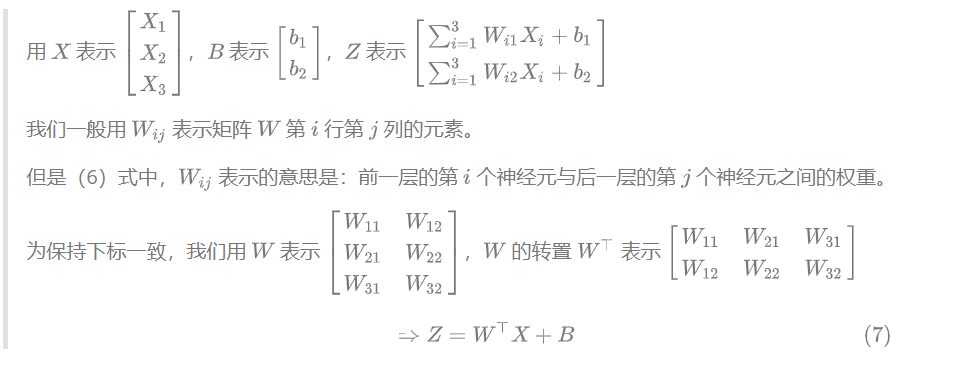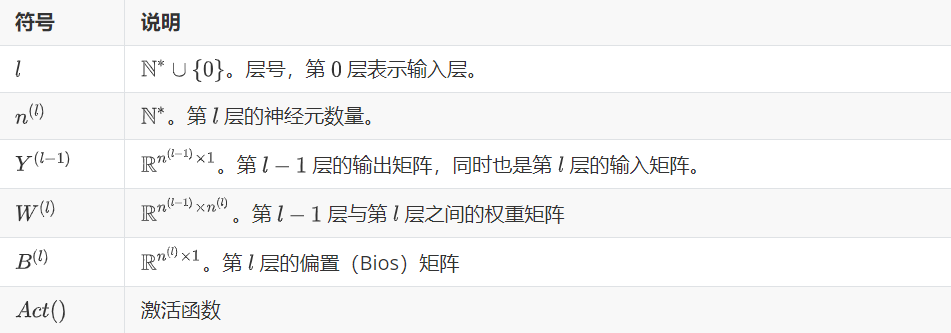$Z^{(l)}=(W^{(l)})^\top Y^{(l-1)}+B^{(l)}$$Y^{(l)}=Act(Z^{(l)})$

## 反向传播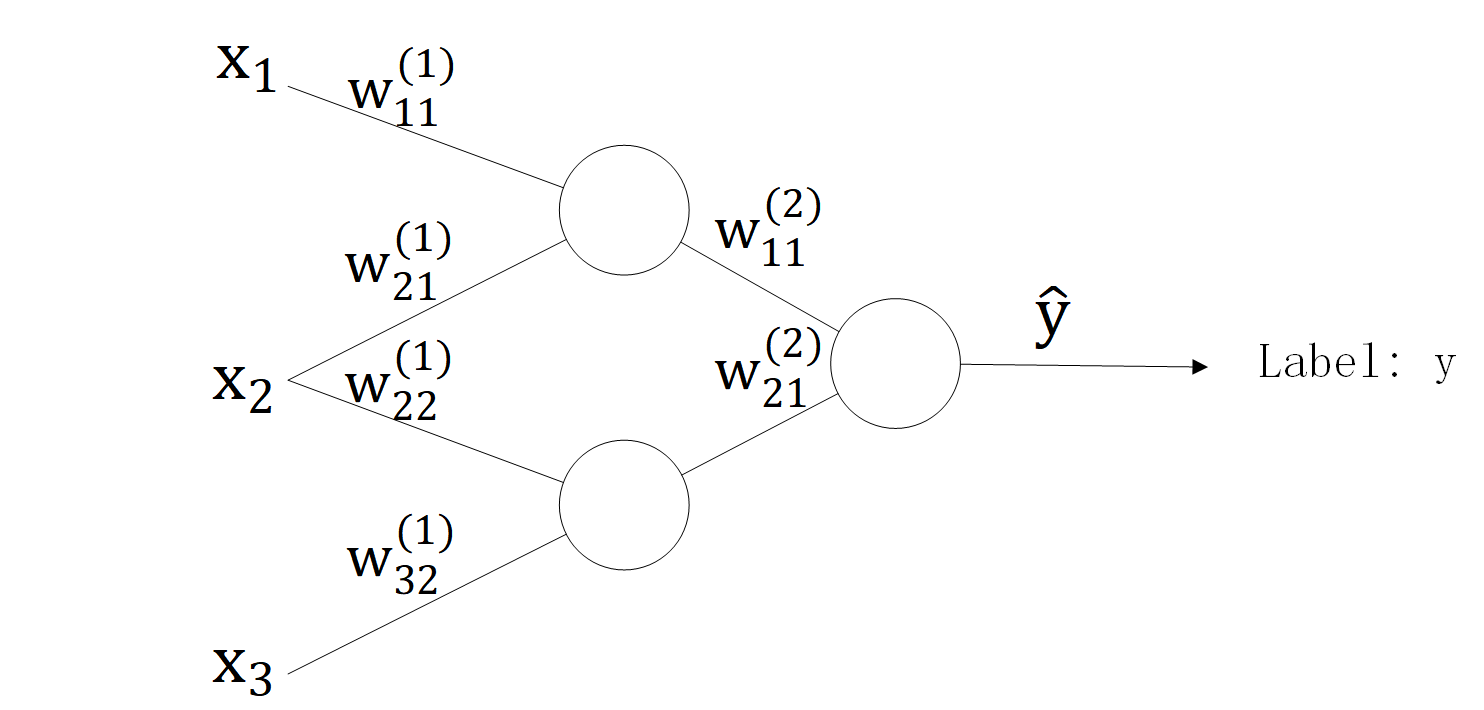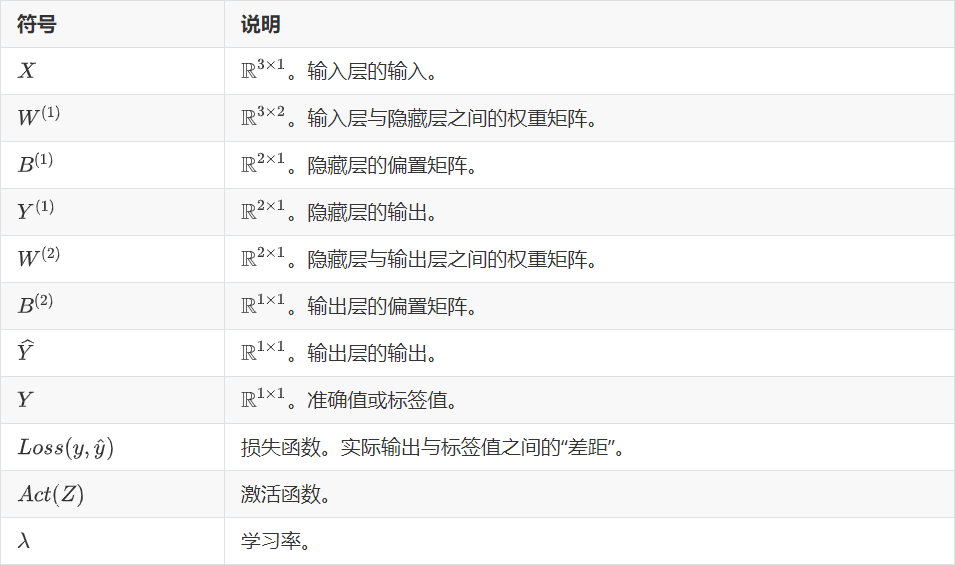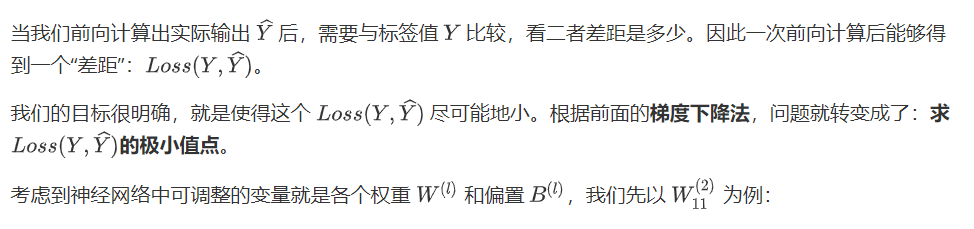### 简单的例子

#### 目标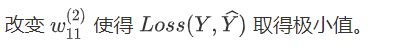#### 方法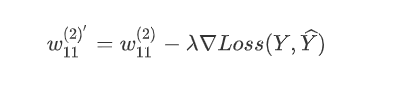## 计算$\nabla Loss(Y,\widehat Y)=\frac{\partial{Loss(Y,\widehat Y)}}{\partial{w^{(2)}_{11}}}$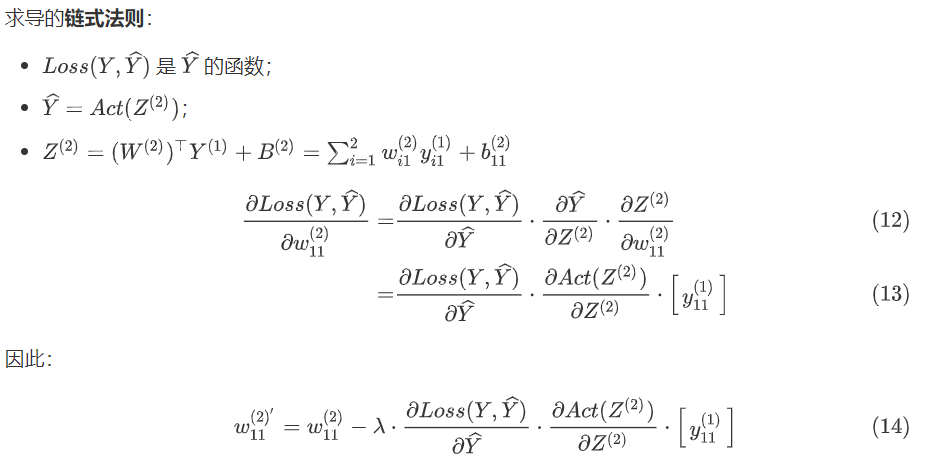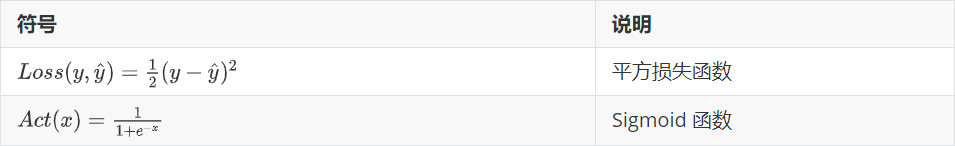$\frac{\partial{Loss(y,\widehat y)}}{\partial{\widehat y}}=-（y-\widehat y）$$\frac{\partial{Act(x)}}{\partial{x}}=Act(x)\cdot(1-Act(x))$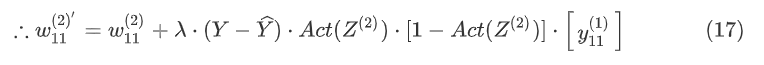$W^{(2)'}=W^{(2)}+\lambda\cdot(Y-\widehat Y)\cdot Act(Z^{(2)})[1-Act(Z^{(2)})]\cdot Y^{(1)}$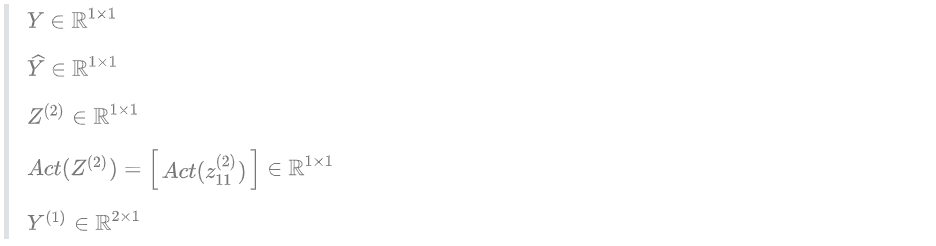### 又一个简单的例子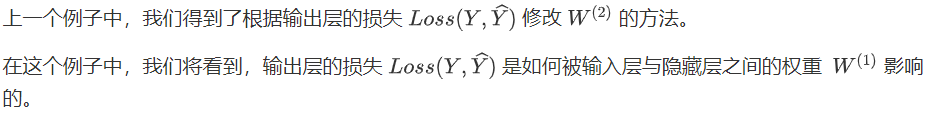$\widehat Y=Act(Z^{(2)})=Act((W^{(2)})^\top Y^{(1)}+B^{(2)})$$Y^{(1)}=Act(Z^{(1)})=Act((W^{(1)})^\top X+B^{(1)})$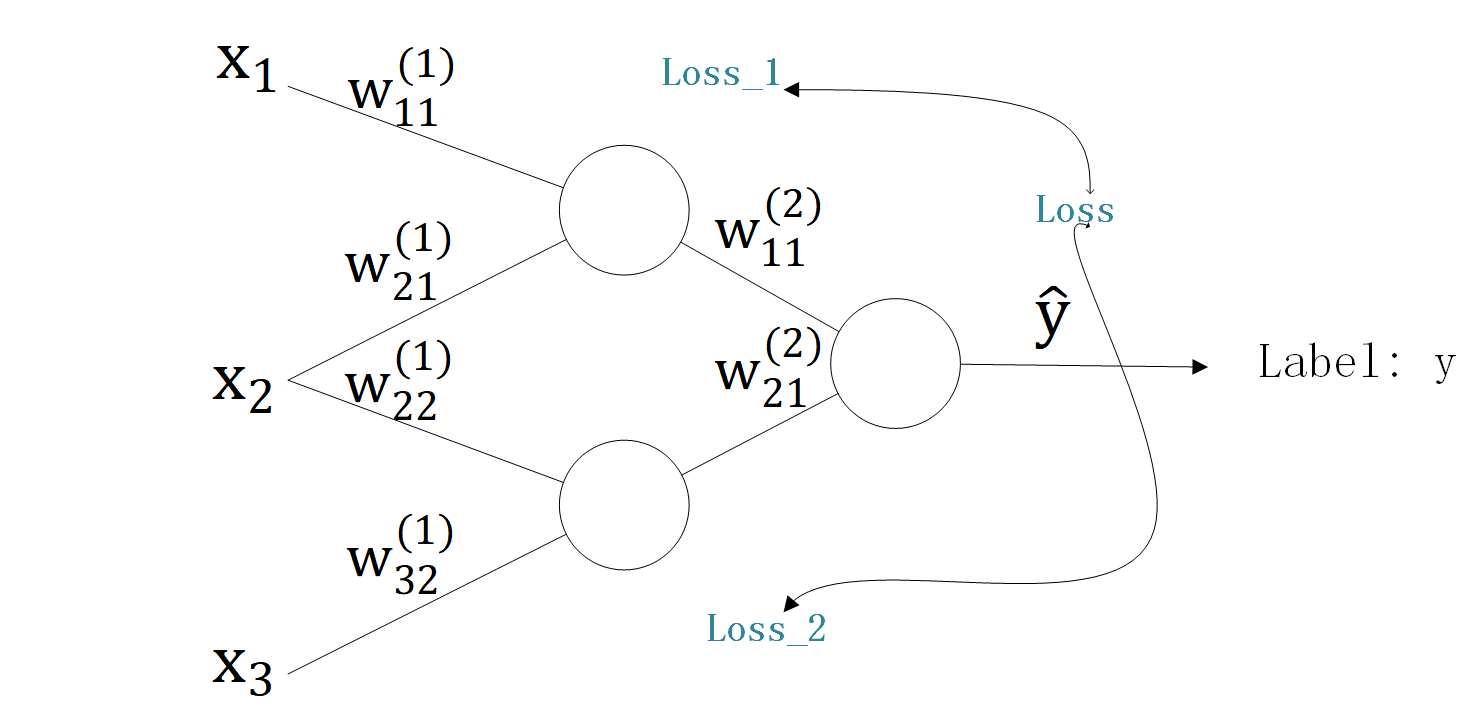$\nabla Loss(Y,\widehat Y)=\frac{\partial{Loss(Y,\widehat Y)}}{\partial{W^{(1)}}}=\frac{\partial{Loss(Y,\widehat Y)}}{\partial{}Z^{(1)}}\cdot\frac{\partial{Z^{(1)}}}{\partial{W^{(1)}}}$$\frac{\partial{Loss(Y,\widehat Y)}}{\partial{Z^{(1)}}}=\frac{\partial{Loss(Y,\widehat Y)}}{\partial{Z^{(2)}}}\cdot\frac{\partial{Z^{(2)}}}{\partial{Y^{(1)}}}\cdot\frac{\partial{Y^{(1)}}}{\partial{Z^{(1)}}}$$\nabla Loss(Y,\widehat Y)=\frac{\partial{Loss(Y,\widehat Y)}}{\partial{W^{(1)}}}$
=\frac{\partial{Loss(Y,\widehat Y)}}{\partial{Z^{(2)}}}\cdot\frac{\partial{Z^{(2)}}}{\partial{Y^{(1)}}}\cdot\frac{\partial{Y^{(1)}}}{\partial{Z^{(1)}}}\cdot\frac{\partial{Z^{(1)}}}{\partial{W^{(1)}}}\$$=\frac{\partial{Loss(Y,\widehat Y)}}{\partial{Z^{(2)}}}\cdot (W^{(2)})^\top\cdot\frac{\partial{Y^{(1)}}}{\partial{Z^{(1)}}}\cdot\frac{\partial{Z^{(1)}}}{\partial{W^{(1)}}}$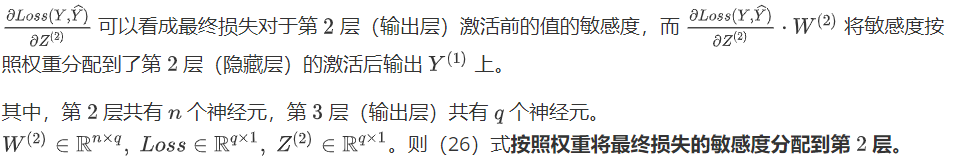$W^{(1)'}$$=W^{(1)}-\lambda\nabla Loss(Y,\widehat Y)$$=W^{(1)}+\lambda\cdot\frac{\partial{Loss(Y,\widehat Y)}}{\partial{Z^{(2)}}}\cdot (W^{(2)})^\top\cdot\frac{\partial{Y^{(1)}}}{\partial{Z^{(1)}}}\cdot\frac{\partial{Z^{(1)}}}{\partial{W^{(1)}}}$$W^{(1)'}=W^{(1)}+\lambda\cdot(Y-\widehat Y)\cdot(W^{(2)})^\top\cdot Act(Z^{(1)})[1-Act(Z^{(1)})]\cdot X$

### 小小的总结$W^{(2)'}=W^{(2)}+\lambda\cdot(Y-\widehat Y)\cdot Act(Z^{(2)})[1-Act(Z^{(2)})]\cdot Y^{(1)}$$W^{(1)'}=W^{(1)}+\lambda\cdot\Big[(Y-\widehat Y)\cdot(W^{(2)})^\top\Big]\cdot Act(Z^{(1)})[1-Act(Z^{(1)})]\cdot X$

## 代码实现

# 定义神经网络类
class neuralNetwork:

# 初始化神经网络
def __init__(self, InputNodes, HiddenNodes, OutputNodes, LearningRate):
# 设置输入层、隐藏层、输出层感知机数量
self.in_nodes = InputNodes
self.hid_nodes = HiddenNodes
self.out_nodes = OutputNodes
# 学习率
self.LR = LearningRate
# 权重矩阵W_ih, W_ho表示Input到Hidden，Hidden到Output的权重矩阵
# 形式如下：
# w_11 w_12
# w_21 w_22
# w_ij表示上一层第i结点到下一层第j结点的权重值
self.W_ih = np.random.normal(0.0, pow(self.hid_nodes, -0.5), (self.in_nodes, self.hid_nodes))
self.W_ho = np.random.normal(0.0, pow(self.out_nodes, -0.5), (self.hid_nodes, self.out_nodes))

# 激活函数为sigmoid函数
self.activate_func = lambda x:scipy.special.expit(x)
self.inv_activate_func = lambda x: scipy.special.logit(x)

# 训练神经网络，输入+目标输出
def train(self, inputs_list, targets_list):
# 将列表输入转换为矩阵
inputs = np.array(inputs_list, ndmin = 2).T
targets = np.array(targets_list, ndmin = 2).T
# 计算隐藏层输入: W_ih·inputs
hidden_inputs = np.dot(self.W_ih.T, inputs)
# 计算隐藏层输出
hidden_outputs = self.activate_func(hidden_inputs)

# 计算输出层输入: W_ho·hidden_outputs
final_inputs = np.dot(self.W_ho.T, hidden_outputs)
# 计算输出层输出
final_outputs = self.activate_func(final_inputs)

# 输出层结点误差errors，误差函数为误差平方和sum(errors^2)
output_errors = targets - final_outputs
# 隐藏层节点误差，通过权重分配
hidden_errors = np.dot(self.W_ho, output_errors)

# 更新隐藏层与输出层之间的权重
self.W_ho += (self.LR * np.dot((output_errors * final_outputs * (1.0 - final_outputs)), hidden_outputs.T)).T

# print((hidden_errors * hidden_outputs * (1.0 - hidden_outputs)).shape)
# 更新输出层与隐藏层之间的权重
self.W_ih += (self.LR * np.dot((hidden_errors * hidden_outputs * (1.0 - hidden_outputs)), inputs.T)).T

# 查询函数，训练完后进行验证
def query(self, inputs_list):
# 将列表输入转为矩阵
inputs = np.array(inputs_list, ndmin = 2).T

# 计算隐藏层输入
hidden_inputs = np.dot(self.W_ih.T, inputs)
# 计算隐藏层输出
hidden_outputs = self.activate_func(hidden_inputs)

# 计算输出层输入
final_inputs = np.dot(self.W_ho.T, hidden_outputs)
# 计算输出层输出
final_outputs = self.activate_func(final_inputs)

return final_outputs


## 后记

（我终于放弃了用 wordpress 文章编辑器编辑 数学公式了இ௰இ，生活太南了）

There are no products in the cart!
Subtotal
¥0.00
Total
¥0.00
Continue Shopping
0